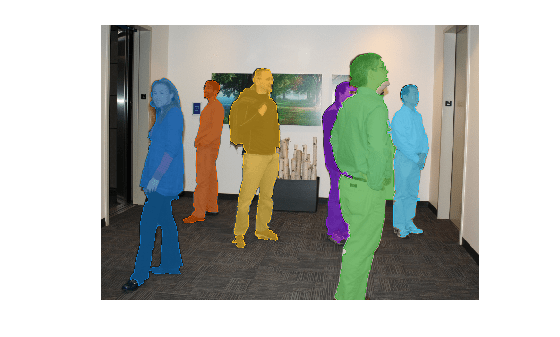Insert masks in image or video stream

## Syntax

``RGB = insertObjectMask(I,BW)``
``RGB = insertObjectMask(I,maskstack)``
``RGB = insertObjectMask(___,Name,Value)``

## Description

example

````RGB = insertObjectMask(I,BW)` inserts a mask `BW` into the specified image `I` and returns the result as a truecolor image `RGB`.```

example

````RGB = insertObjectMask(I,maskstack)` inserts a set of masks `maskstack` into the specified image `I` and returns the result as a truecolor image `RGB`.```
````RGB = insertObjectMask(___,Name,Value)` specifies options using one or more name-value pair arguments in addition to the input arguments in previous syntaxes.```

## Examples

collapse all

Read an image into the workspace.

`I = imread('visionteam1.jpg');`

`load('visionteam1Maskstack.mat')`

Insert the masks into the image.

`RGB = insertObjectMask(I,maskstack,'LineColor','white','LineWidth',2);`

Display the image with the masks inserted.

```figure imshow(RGB)```Read an image into the workspace.

`I = imread('visionteam1.jpg');`

`load('visionteam1Maskstack.mat');`

Insert the masks into the image, specifying a unique color for each mask.

```numMasks = size(maskstack,3); RGB = insertObjectMask(I,maskstack,'Color',lines(numMasks));```

Display the image with the inserted masks.

```figure imshow(RGB)```## Input Arguments

collapse all

Input image, specified as a truecolor (RGB) image, m-by-n-by-3 array, or a grayscale image, m-by-n array.

Data Types: `single` | `double` | `int16` | `uint8` | `uint16`

Input mask image, specified as an m-by-n logical matrix.

Data Types: `logical`

Stack of mask images, specified as an m-by-n-by-P logical array, where P is the total number of masks in the stack.

`maskstack` has the same width and height as `I`.

Data Types: `logical`

### Name-Value Arguments

Specify optional comma-separated pairs of `Name,Value` arguments. `Name` is the argument name and `Value` is the corresponding value. `Name` must appear inside quotes. You can specify several name and value pair arguments in any order as `Name1,Value1,...,NameN,ValueN`.

Example: ```RGB = insertObjectMask(I,maskstack, 'LineColor','white','LineWidth',2);```

Color of each mask, specified as the comma-separated pair consisting of `'Color'` and one of these values:

• P-by-3 matrix of RGB triplets, where P is the total number of masks

• P-element vector of MATLAB® ColorSpec names

• 1-by-3 RGB triplet or scalar MATLAB ColorSpec name, specifying the color to use for all masks

Data Types: `single` | `double` | `int8` | `int16` | `int32` | `int64` | `uint8` | `uint16` | `uint32` | `uint64` | `logical` | `char` | `string`

Opacity of mask, specified as the comma separated pair consisting of `'Opacity'` and a scalar value in the range [0 1]. The value `1` makes the mask completely opaque and the value `0` makes the mask completely transparent.

Data Types: `single` | `double` | `int8` | `int16` | `int32` | `int64` | `uint8` | `uint16` | `uint32` | `uint64`

Color of mask borders, specified as the comma separated pair consisting of `'LineColor'` and one of these values:

• `'auto'``'LineColor'` uses the same value or values as `'Color'`.

• P-by-3 matrix of RGB triplets, where P is the total number of masks

• P-element vector of MATLAB ColorSpec names

• 1-by-3 RGB triplet or scalar MATLAB ColorSpec name, specifying the color to use for all masks

Data Types: `single` | `double` | `int8` | `int16` | `int32` | `int64` | `uint8` | `uint16` | `uint32` | `uint64` | `logical` | `char` | `string`

Opacity of the mask borders, specified as the comma-separated pair consisting of `'LineOpacity'` and a scalar value in the range of [0 1].

Data Types: `single` | `double` | `int8` | `int16` | `int32` | `int64` | `uint8` | `uint16` | `uint32` | `uint64`

Width of mask borders, specified as the comma-separated pair consisting of `'LineWidth'` and a positive scalar. Specify `'LineWidth'` in pixel units.

Data Types: `single` | `double` | `int8` | `int16` | `int32` | `int64` | `uint8` | `uint16` | `uint32` | `uint64`

## Output Arguments

collapse all

Output image, returned as a truecolor image of class `uint8`, with the same m-by-n dimensions as `I`.

## Tips

• When masks overlap and the same pixel is in more than one mask, `maskstack(:,:,i)` takes precedence over `maskstack(:,:,j)`, where `i` < `j`.

• For better performance, set `'LineOpacity'` to `0` to disable drawing the edges.